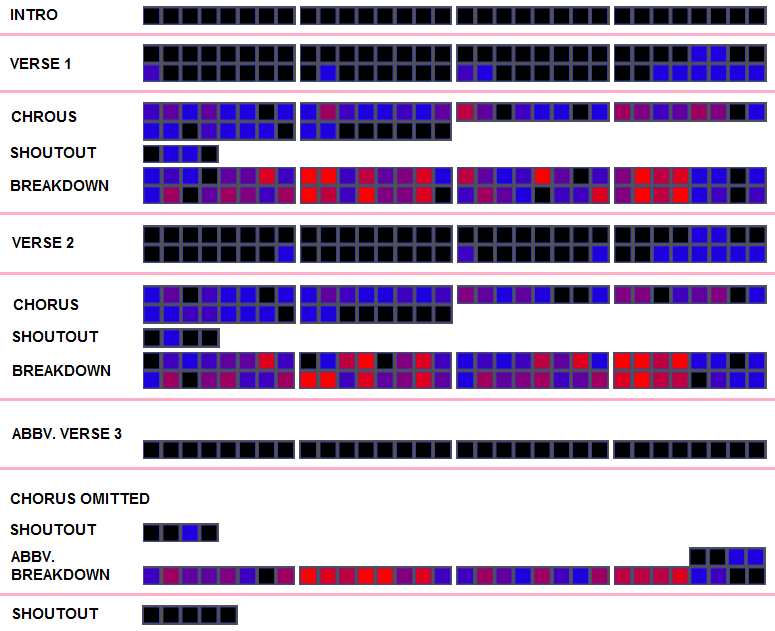## Archive for October, 2012

### extrinsic bias in the prediction market

People have proposed using price signals from prediction markets to estimate the odds of certain events. On Intrade right now, you can buy contracts for the two outcomes of the 2012 US Presidential Election. Each contract expires at $10 if the event occurs or$0 if it doesn’t. For example, “Barack Obama wins” contracts are $6.33 a pop right now, while “Mitt Romney wins” contracts go for$3.65. On the page, these are taken directly as probabilities, because it is assumed that the gamble is zero-sum.

Specifically, if $$p$$ and $$\bar{p}=1-p$$ are respectively the probabilities of two complementary events, and $$a$$ and $$b$$ are respectively the prices of contracts on them, which can be bought and sold freely, then no-arbitrage imposes that $$-a-b+10 = 0$$ and statistical no-arbitrage imposes $$-\bar{p}a +p(10-a) = 0$$ and $$-pb +\bar{p}(10-b) = 0$$. Solving indeed gives the prices $$a=10p$$ and $$b=10\bar{p}$$.

However, this isn’t the end of the story.

### gangnam style analysis

This brilliant infinite looping version of Gangnam Style uses machine learning to join the similar points of the song to each other, and allows branching among them. It is slightly annoying, however, because the song does not start on the first beat, so all the bars are misaligned by one. I decided to redo the chart with the correct alignment and labeling, so here it is:For any non-negative random variable $$X$$ having finite first and second moments, $$\mathbb P(X>0) \ge (\mathbb EX)^2/\mathbb EX^2$$.